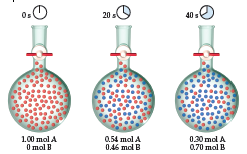# Problem: If the experiment below is run for 60 s, 0.16 mol A remain. Which of the following statements is or are true?After 60 s there are 0.84 mol B in the flask.The decrease in the number of moles of A from t1 = 0 to t2 = 20 s is greater than that for t1 = 40 to t2 = 60 s.The average rate for the reaction from t1 = 40 to t2 = 60 s is 7.0 10-3 M/s.

🤓 Based on our data, we think this question is relevant for Professor Tang's class at USF.

###### FREE Expert Solution
• Analyzing each statement, for Statement I: after 60 s there are 0.84 mol B in the flask

• Checking the diagrams provided, each flask showed that sum of mole A and B, regardless of the time, will always be 1. Where at: 0s, moles A = 1 mol;

20s, moles A = 0.54 moles and moles B = 0.46 moles (0.54 + 0.46 = 1)

40s, moles A = 0.30 moles and moles B = 0.70 moles (0.30 + 0.70 = 1)

• Therefore, at 60s if A is 0.16 moles, then B must be 0.84 moles (1 - 0.16 = 0.84 moles)

Statement I is true###### Problem Details

If the experiment below is run for 60 s, 0.16 mol A remain. Which of the following statements is or are true?1. After 60 s there are 0.84 mol B in the flask.
2. The decrease in the number of moles of A from t1 = 0 to t2 = 20 s is greater than that for t1 = 40 to t2 = 60 s.
3. The average rate for the reaction from t1 = 40 to t2 = 60 s is 7.0 10-3 M/s.